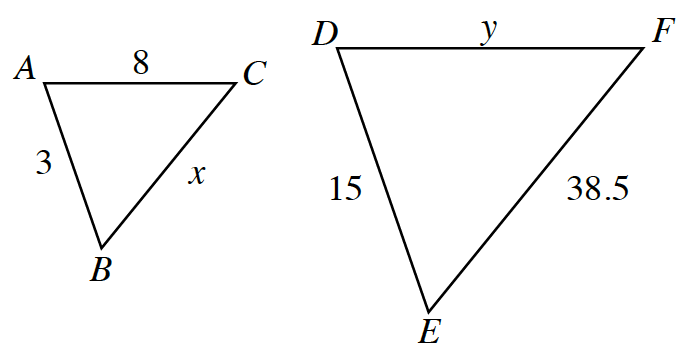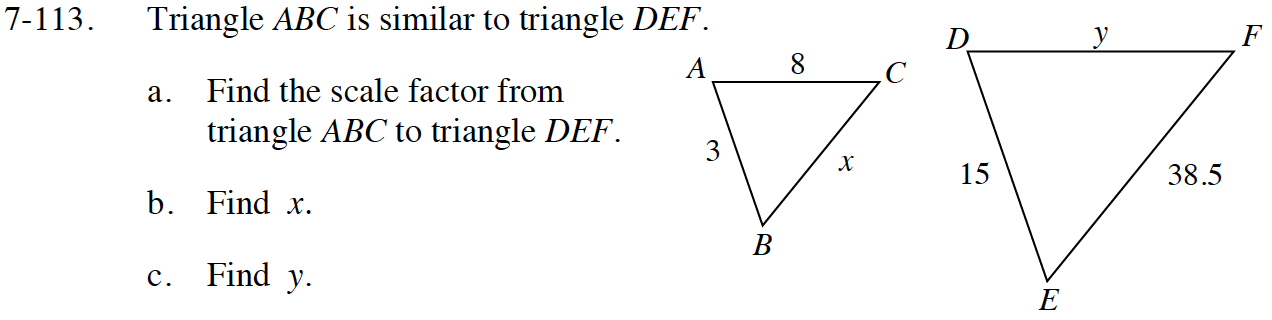Home > ACC7 > Chapter cc27 > Lesson cc27.2.2 > Problem7-113

7-113.
1.Triangle ABC is similar to triangle DEF. Homework Help ✎

1. Find the scale factor from triangle ABC to triangle DEF.

2. Find x.

3. Find y.Find the scale factor by using two corresponding sides.

The scale factor is 5.

Divide by the scale factor.

$x=\frac{38.5}{5}$

Multiply the corresponding side by the scale factor.